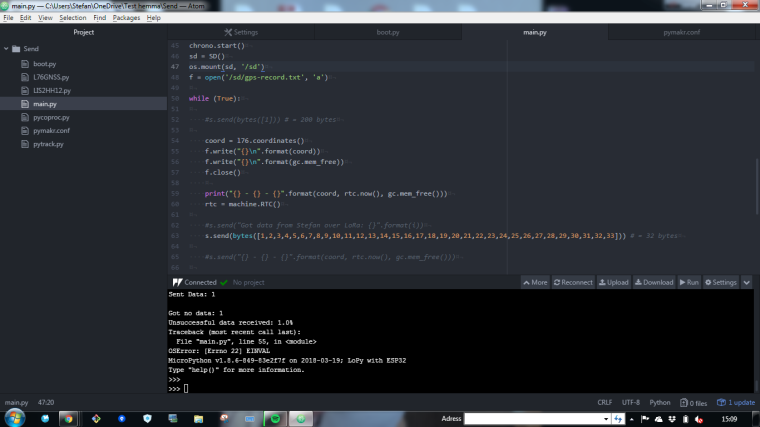# SD Card - Store GPS GPS-coordinates to SD card

• Im did try this and it works fine

``````from machine import SD
import os
import gc

print('Hej')
gc.enable()

sd = SD()
os.mount(sd, '/sd')

while True:

f = open('/sd/test.txt', 'a')
f.write('Testing SD card write operations')
f.write('\n')
#gc.collect()
f.write('Does this work')
#gc.collect()
f.close()
``````

When I try this exampel, I dont get anything, I dont understand how I should pass on the data into the sd card (data and coordinates).
Sorry the look of the post:

``````import machine
import math
import network
import os
import time
import utime
import socket
import pycom
import gc
from machine import RTC
from machine import SD
from machine import Timer
from L76GNSS import L76GNSS
from pytrack import Pytrack
from network import LoRa
# setup as a station

pycom.heartbeat(False)
for cycles in range(1): # stop after 1 cycles
time.sleep(0)

lora = LoRa(mode=LoRa.LORA,tx_power = 14, sf = 7, frequency=863000000)
s = socket.socket(socket.AF_LORA, socket.SOCK_RAW)
s.setblocking(False)

gc.enable()

i = 0
failed = 0
counter_sent = 0
counter_received = 0

# setup rtc
rtc = machine.RTC()
rtc.ntp_sync("pool.ntp.org")
utime.sleep_ms(750)
print('\nRTC Set from NTP to UTC:', rtc.now())
utime.timezone(7200)
print('Adjusted from UTC to EST timezone', utime.localtime(), '\n')

py = Pytrack()
l76 = L76GNSS(py, timeout=0.4)

chrono = Timer.Chrono()
chrono.start()
sd = SD()
os.mount(sd, '/sd')
f = open('/sd/gps-record.txt', 'w')

while (True):

#s.send(bytes()) # = 200 bytes

coord = l76.coordinates()
f.write("{} - {}\n".format(coord, rtc.now()))

print("{} - {} - {}".format(coord, rtc.now(), gc.mem_free()))
rtc = machine.RTC()

#s.send("Got data from Stefan over LoRa: {}".format(i))
s.send(bytes([1,2,3,4,5,6,7,8,9,10,11,12,13,14,15,16,17,18,19,20,21,22,23,24,25,26,27,28,29,30,31,32,33])) # = 32 bytes

#s.send("{} - {} - {}".format(coord, rtc.now(), gc.mem_free()))

data = s.recv(64)
f.write("{}\n".format(data))
print(data)
counter_sent+=1
print('Sent Data: {}'.format(counter_sent))
if data:
print(data)
print('Received Data: {}'.format(data))
counter_received+=1
print('Received Data: {}'.format(counter_received))
print('Successful data received: {}%'.format(counter_received/counter_sent))
pycom.rgbled(0x007f00) # green
print(' ')
else:
print(' ')
failed +=1
print('Got no data: {}'.format(failed))
print('Unsuccessful data received: {}%'.format(failed/counter_sent))
pycom.rgbled(0x7f0000)
#time.sleep(0.4)
``````• Also you have not checked if `coord = l76.coordinates()` return something it can contain `None`

• @stefan85e
what is your current code? Because attached is different than on screenshot from pymakr.
In pymakr you have an error because you open file before loop
but in the loop you close file and in another iteration it can not acces it and then you got an error on line `55`

look at `f.flush()`